# Samacheer Kalvi 10th Maths Solutions Chapter 3 Algebra Ex 3.12

## Tamilnadu Samacheer Kalvi 10th Maths Solutions Chapter 3 Algebra Ex 3.12

10th Maths Exercise 3.12 Samacheer Kalvi Question 1.
If the difference between a number and its reciprocal is $$\frac{24}{5}$$, find the number.
Solution:
Let a number be x.
Its reciprocal is $$\frac{1}{x}$$
$$x-\frac{1}{x}=\frac{24}{5}$$
$$\frac{x^{2}-1}{x}=\frac{24}{5}$$
5x2 – 5 -24x = 0 ⇒ 5x2 – 24x – 5 = 0
5x2 – 25x + x – 5 = 0
5x(x – 5) + 1 (x – 5) = 0
(5x + 1)(x – 5) = 0
x = $$\frac{-1}{5}$$, 5
∴ The number is $$\frac{-1}{5}$$ or 5.

10th Maths Exercise 3.12 Solution Question 2.
A garden measuring 12m by 16m is to have a pedestrian pathway that is ‘w’ meters wide installed all the way around so that it increases the total area to 285 m2. What is the width of the pathway?
Solution:
Area of ABCD = 16 × 12 2
= 192 m2
Area of A’B’C’D’ (12 + 2w)(16 + 2w)
192 + 32 w + 24 w + 4 w2 = 285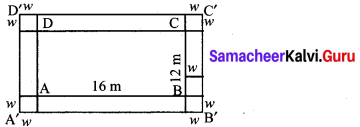4w2 + 56w – 93 = 0
4w2 + 62w – 6w – 93 = 0
2w(2w + 31) – 3(2w + 31) = 0
(2w – 3)(2w + 31) = 0
w = 1.5 or $$\frac{-31}{2}$$ = 15.5
w = – 15.5 cannot possible 3
∴ w = $$\frac{3}{2}$$ = 1.5 m
(w cannot be (-ve))
The width of the pathway = 1.5 m.

Ex 3.12 Class 10 Samacheer Question 3.
A bus covers a distance of 90 km at a uniform speed. Had the speed been 15 km/hour more it would have taken 30 minutes less for the journey. Find the original speed of the bus.
Solution:
Let x km/hr be the constant speed of the bus.
The time taken to cover 90 km = $$\frac{90}{x}$$ hrs.
When the speed is increased bus 15 km/hr.
= $$\frac{90}{x+15}$$
It is given that the time to cover 90 km is reduced by $$\frac{1}{2}$$ hrs.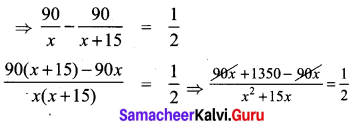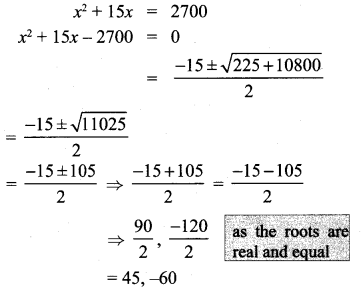The speed of the bus cannot be -ve value.
∴ The original speed of the bus is 45 km/hr.

Exercise 3.12 Class 10 Samacheer Question 4.
A girl is twice as old as her sister. Five years hence, the product of their ages (in years) will be 375. Find their present ages.
Solution:
Let the age of the girl be = 2y years
Her sister’s age is = y years
(2y + 5)(y + 5) = 375
2y2 + 5y+ 10y + 25 – 375 = 0
2y2 + 15y – 350 = 0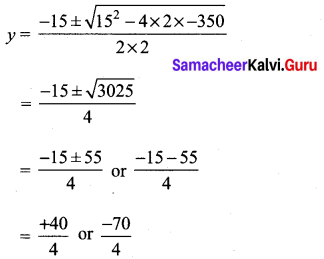y = 10, y cannot be (-ve).
∴ Girls age is 2y = 20 years.
Her sister’s age = y = 10 years.

10th Maths Exercise 3.12 Question 5.
A pole has to be erected at a point on the T boundary of a circular ground of diameter j 20 m in such a way that the difference of its i distances from two diametrically opposite j fixed gates P and Q on the boundary is 4 m. Is i it possible to do so? If answer is yes at what j distance from the two gates should the pole j be erected?
Solution:
PQ = 20 m
PX – XQ = 4 m …………… (1)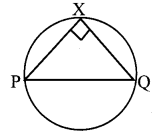Squaring both sides,
PX2 + XQ2 – 2PX . QX = 16 (∵ ∠Q × p = 90°)
PQ2 – 2P × QX = 16
400 – 16 = 2PX × QX
384 = 2PX – QX
PX . QX = 192
∴ (PX + QX)2 = PX2 + QX2 + 2PX . QX
= 400 + 2 × 192
= 784 = 282
∴ PX + QX = 28
From (1) & (2) 2PX = 32 ⇒ PX = 16 m QX = 12 m
∴Yes, the distance from the two gates to the pole PX and QX is 12 m, 16m.

Samacheer Kalvi Guru 10th Maths Question 6.
From a group of black bees 2x2, square root of half of the group went to a tree. Again eight- ninth of the bees went to the same tree. The remaining two got caught up in a fragrant lotus. How many bees were there in total?
Solution:
Total no. of bees = 2x2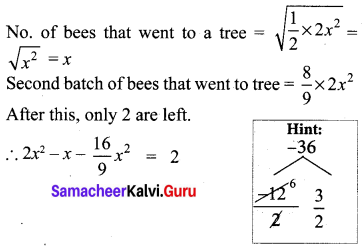18x2 – 9x – 16x2 = 2 × 9
2x2 – 9x – 18 = 0
(x – 6)(2x + 3) = 0
x = 6, x = $$\frac{-3}{2}$$ (it is not possible)
No. of bees in total = 2x2
= 2 × 62 = 72

10th Maths Algebra Exercise 3.12 Question 7.
Music is been played in two opposite galleries with certain group of people. In the first gallery a group of 4 singers were singing and in the second gallery 9 singers were singing. The two galleries are separated by the distance of 70 m. Where should a person stand for hearing the same intensity of the singers voice? (Hint: The ratio of the sound intensity is equal to the square of the ratio of their corresponding distances).
Solution:
Let the person stand at a distance ‘d’ from 2nd gallery having 9 singers.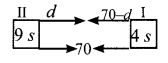Given that ratio of sound intensity is equal to the square of the ratio of their corresponding distance.
∴ $$\frac{9}{4}=\frac{d^{2}}{(70-d)^{2}}$$
4d2 = 9(70 – d)2
4d2 = 9(702 – 140d + d2)
4d2 = 9 × 702 – 9 × 140d + 9d2
∴ 5d2 – 9 × 140d + 9 × 702 = 0
5d2 = 1260d + 44100 = 0
d2 – 252d + 8820 = 0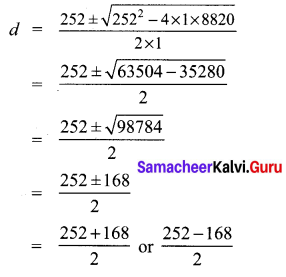= $$\frac{420}{2} \text { or } \frac{84}{2}$$
= 120 or 42
∴ The person stand at a distance 28m from the first and 42 m from second gallery.

Samacheer Kalvi.Guru 10th Maths Question 8.
There is a square field whose side is 10 m. A square flower bed is prepared in its centre leaving a gravel path all round the flower bed. The total cost of laying the flower bed and gravelling the path at ₹ 3 and ₹ 4 per square metre respectively is ₹364. Find the width of the gravel path.
Solution: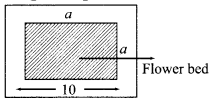Area of the flower bed = a2
Area of the gravel path = 100 – a2
Area of total garden =100
given cost of flower bed + gravelling = ₹ 364
3a2 + 4 (100 – a2) = ₹ 364
3a2 + 400 – 4a2 = 364
∴ a2 = 400 – 364
= 36 ⇒ a = 6
width of gravel path =$$\frac{10-6}{2}=\frac{4}{2}$$ = 2 cm

10th Maths Exercise 3.12 9th Sum Question 9.
Two women together took 100 eggs to a market, one had more than the other. Both sold them for the same sum of money. The first then said to the second: “If I had your eggs, I would have earned ₹ 15”, to which the second replied: “If I had your eggs, I would have earned ₹ 6 $$\frac { 2 }{ 3 }$$”. How many eggs did each had in the beginning? Answer:
Number of eggs for the first women be ‘x’
Let the selling price of each women be ‘y’
Selling price of one egg for the first women = $$\frac { y }{ 100-x }$$
By the given condition
(100 – x) $$\frac { y }{ x }$$ = 15 (for first women)
y = $$\frac { 15 }{ 100-x }$$ ……(1)
x × $$\frac { y }{ (100-x) }$$ = $$\frac { 20 }{ 3 }$$ [For second women]
y = $$\frac { 20(100-x) }{ 3x }$$ ……..(2)
From (1) and (2) We get
$$\frac { 15 }{ 100-x }$$ = $$\frac { 20(100-x) }{ 3x }$$
45x2 = 20(100 – x)2
(100 – x)2 = $$\frac{45 x^{2}}{20}$$ = $$\frac { 9 }{ 4 }$$ x2
∴ 100 – x = $$\sqrt{\frac{9}{4} x^{2}}$$
100 – x = $$\frac { 3x }{ 2 }$$
3x = 2(100 – x)
3x = 200 – 2x
3x + 2x = 200 ⇒ 5x = 200
x = $$\frac { 200 }{ 5 }$$ ⇒ x = 40
Number of eggs with the first women = 40
Number of eggs with the second women = (100 – 40) = 60

10th Maths Exercise 3.12 In Tamil Question 10.
The hypotenuse of a right-angled triangle is 25 cm and its perimeter 56 cm. Find the length of the smallest side.
Solution: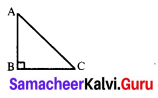AB + BC + CA = 56 cm
AC = 25 cm
AB + BC = 56 – 25 = 31
AB2 + BC2 = AC2
(AB + BC)2 – 2AB . BC = AC2 [∵ a2 + b2 = (a + b)2 – 2ab]
312 – 2AB . BC = 252
-2AB . BC = 625 – 961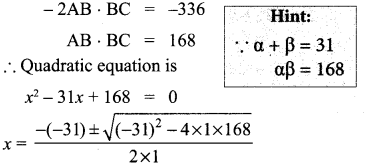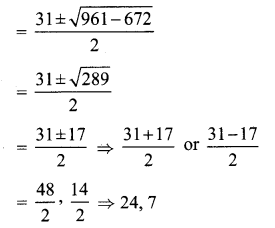∴ The length of the smallest side is 7 cm.